# How to simulate missing values in Python?¶

Thomas Schmitt, Sept. 2019

## Intro¶

Assessing the performance of a model which deals with missing values requires to be able to generate such missing entries in the data, in order to have ground truth information.

In this notebbok we consider three ways to ampute a dataset, which means to replace some values with np.nan.

In :
# first let's generate the complete data

import numpy as np
import matplotlib.pyplot as plt
import seaborn as sns
%matplotlib inline

np.random.seed(0)  # fix the seed

n_samples = 900

# Generate normal data with no covariance.
mean = (0,0)
cov = [[1,0],[0,1]]
X_complete = np.random.multivariate_normal(mean, cov, size=n_samples)
# X_complete = np.random.uniform(0, 1, size=(n_samples,2))

sns.jointplot(X_complete[:,0], X_complete[:,1], label = 'Complete data');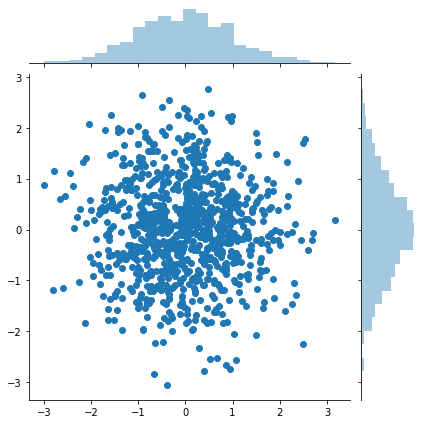# Amputation¶

We want to simulate a matrix X_obs from X_complete with missing entries (remplacing some entries by np.nan).
To do so we sample a missing pattern matrix M with same shape as X_complete indicating missing entries:

$X_{obs} = X_{complete} \cdot \mathbb{1}_{\{M=0\}} + \mbox{np.nan} \cdot \mathbb{1}_{\{M=1\}}$

The mechanisms generating missing values can be various but usually they are classified into three main categories defined by Rubin 1976

1. Missing Completly at Random (MCAR)
2. Missing at Random (MAR)
3. Missing Not at Random (MNAR)

The first two are also qualified as ignorable missing values mechanisms, for instance in likelihood-based approaches to handle missing values, whereas the MNAR mechanism generates nonignorable missing values.

## 1. MCAR Mechanism (Missing Completly at Random)¶

Values are Missing Completely At Random (MCAR) if the missingness pattern is independent of the values (observed or not). The probability of being missing does not depend on the data (neither $X_{obs}$ nor $X_{miss}$, where $X_{miss}$ correponds to the missing values.). Formally:

$\mathbb{P} (M | X_{obs},X_{miss})= \mathbb{P}(M)\ \forall X_{obs},\ \forall X_{miss}$
i.e. $M \perp \! \! \! \perp X_{complete}$

In Python, the easiest way is to sample a Bernouilli to mask some data point

In :
def ampute_mcar(X_complete, missing_rate = .2):
# Mask completly at random some values
M = np.random.binomial(1, missing_rate, size = X_complete.shape)
X_obs = X_complete.copy()
print('Percentage of newly generated mising values: {}'.\
format(np.sum(np.isnan(X_obs))/X_obs.size))
return X_obs

In :
X_obs_mcar = ampute_mcar(X_complete)

Percentage of newly generated mising values: 0.1922222222222222

In :
print('X_obs_mcar = ')
print(X_obs_mcar[:10])
print('  ...')

X_obs_mcar =
[[ 1.76405235         nan]
[ 0.97873798  2.2408932 ]
[ 1.86755799 -0.97727788]
[        nan         nan]
[-0.10321885  0.4105985 ]
[ 0.14404357  1.45427351]
[ 0.76103773  0.12167502]
[ 0.44386323  0.33367433]
[        nan -0.20515826]
[        nan         nan]]
...

In :
plt.figure(figsize=(5,5))
plt.matshow(X_obs_mcar[:100], aspect='auto', fignum=False)
plt.title('X_obs_mcar');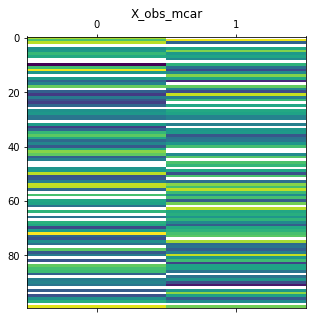In :
# More advanced ploting functions with seaborn
# scripts could be found at: https://github.com/TwsThomas/website/tree/master/static/how-to/python/generate
from utils_plot import (hist_plot, scatter_plot_obs,
scatter_plot_with_missing_completed)

In :
print('Data with "MCAR" mechanism.')
scatter_plot_obs(X_obs_mcar)

Data with "MCAR" mechanism.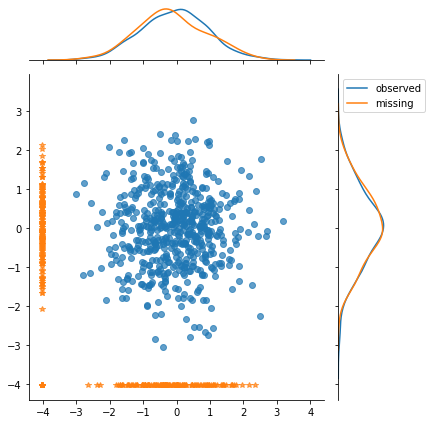In :
print('Plot completed with X_complete information.')
scatter_plot_with_missing_completed(X_obs_mcar, X_complete)

Plot completed with X_complete information.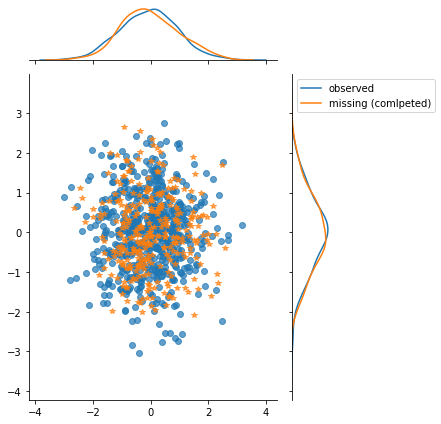In :
hist_plot(X_obs_mcar, X_complete)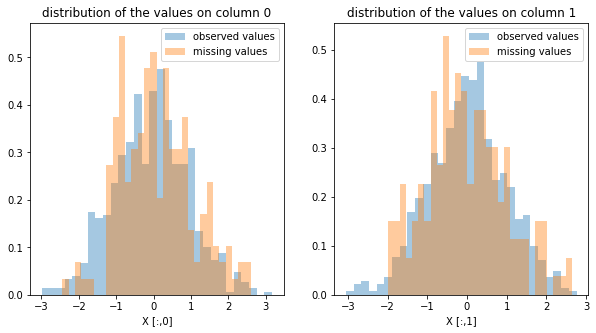From plots and histogram below, we can infer that missingness is independant on the observed and missing values.

In [ ]:



## 2. MAR (Missing At Random)¶

The values are said to be Missing At Random (MAR) if the probability that an entry is missing only depends on the observed values X_obs. Formally,

$\mathbb{P} (M | X\_{obs},X\_{miss})= \mathbb{P}(M|X\_{obs}), \forall X\_{miss}$
i.e. $M \perp \! \! \! \perp X_{miss} \ |\ X\_{obs}$

In :
from sklearn.preprocessing import normalize

def ampute_mar(X_complete, missing_rate=.2, W=None):
""" Observed values censor the missing ones

The proba of being missing: M_proba = X_obs.dot(W)
So for each sample, some observed feature (P=1) will influence
the missingness of some others features (P=0).

e.g. during a questionnary, those who said being busy (X_obs[:,0] = 1)
usualy miss to fill the last question (X_obs[:,-1] = np.nan)
So here W[0,-1] = 1
"""
X_obs = X_complete.copy()
M_proba = np.zeros(X_obs.shape)

if W is None:
# generate the weigth matrix W
W = np.random.randn(X_complete.shape, X_complete.shape)

# Severals iteration to have room for high missing_rate
for i in range(X_obs.shape*2):
# Sample a pattern matrix P
# P[i,j] = 1 will correspond to an observed value
# P[i,j] = 0 will correspond to a potential missing value
P = np.random.binomial(1, .5, size=X_complete.shape)

# potential missing entry do not take part of missingness computation
X_not_missing = np.multiply(X_complete,P)

sigma = np.var(X_not_missing)
M_proba_ = np.random.normal(X_not_missing.dot(W), scale = sigma)

# not missing should have M_proba = 0
M_proba_ = np.multiply(M_proba_, 1-P)  # M_proba[P] = 0

M_proba += M_proba_

thresold = np.percentile(M_proba.ravel(), 100 * (1 - missing_rate))
M = M_proba > thresold

print('Percentage of newly generated mising values: {}'.\
format(np.sum(np.isnan(X_obs))/X_obs.size))
return X_obs

In :
W = np.array([[0,10],[0,0]])
# With this weight matrix W,
# missingness of X[:,1] depends on X[:,0] values
X_obs_mar = ampute_mar(X_complete, W=W)

Percentage of newly generated mising values: 0.2

In :
print('Data with "MAR" mechanism.')
scatter_plot_obs(X_obs_mar)

Data with "MAR" mechanism.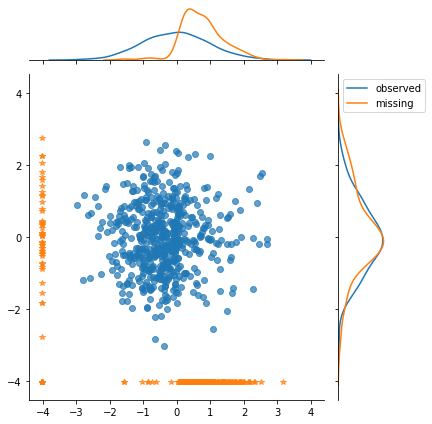From the scatter plot we see that the missing values with nan on the X[:,1] coordinate (y-axis), which are represented as orange star at the bottom, are highly correlated to the X[:,0] values (x-axis).

X[i,0] > .5 => X[i,1] = np.nan with high probability.

In :
print('Samples completed with X_complete information.')
scatter_plot_with_missing_completed(X_obs_mar, X_complete)

Samples completed with X_complete information.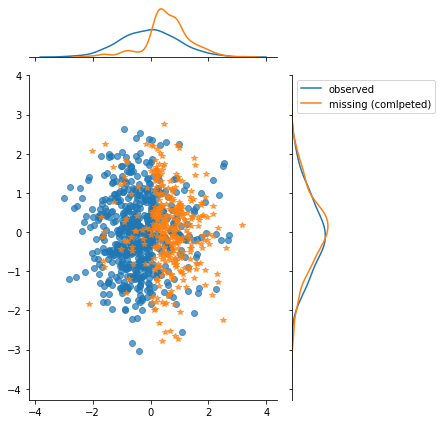In :
hist_plot(X_obs_mar, X_complete)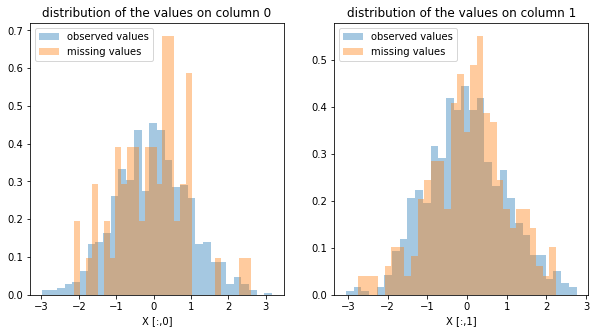Histogram are overlaping : missingness does not depends on the missing values.

# MNAR (Missing Not At Random)¶

Otherwise, if neither MCAR nor MAR, values are Missing Not At Random (MNAR) $\mathbb{P} (M | X\_obs, X\_mis)= \mathbb{P}(M|X\_obs), \forall X\_mis$

Here we ampute the ighest values with highest probability. It might be called "censoring"

In :
from scipy.special import expit as sigmoid  # logistic function

def ampute_mnar(X_complete, missing_rate= .2):
""" ampute X_complete with censoring (Missing Not At Random)

The missingness depends on the values.
This will tends to "censor" X[i,j] where X[i,j] is high
comparing to its column X[:,j]
"""

# M depends on X_complete values
M_proba = np.random.normal(X_complete)
M_proba = normalize(M_proba, norm='l1')

# compute thresold wrt missing_rate
thresold = np.percentile(M_proba.ravel(), 100 * (1- missing_rate))
M = M_proba > thresold

X_obs = X_complete.copy()
print('Percentage of newly generated mising values: {}'.\
format(np.sum(np.isnan(X_obs))/X_obs.size))
return X_obs

In :
X_obs_mnar = ampute_mnar(X_complete)

Percentage of newly generated mising values: 0.2

In :
scatter_plot_obs(X_obs_mnar)
print('X_obs with "MNAR" mechanism')

X_obs with "MNAR" mechanism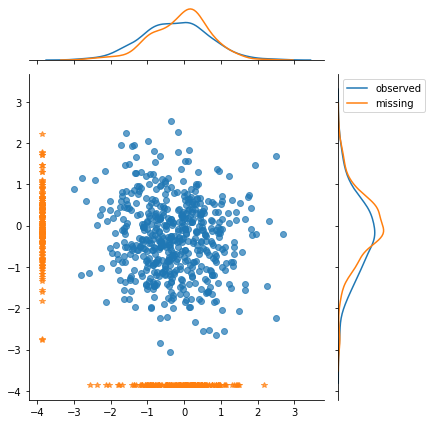In :
print('Samples completed with X_complete information.')
scatter_plot_with_missing_completed(X_obs_mnar, X_complete)

Samples completed with X_complete information.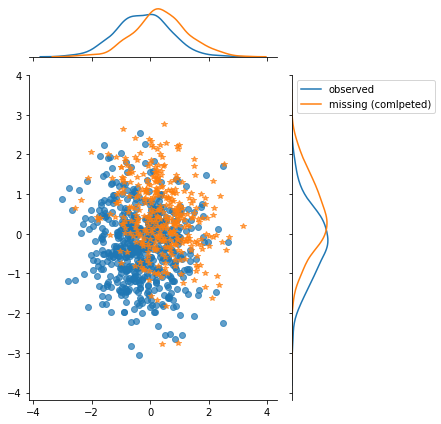In :
hist_plot(X_obs_mnar, X_complete)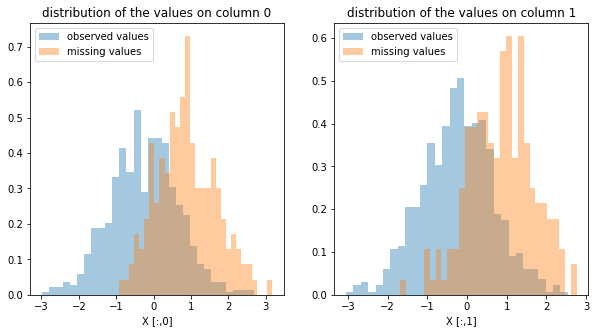The distribution of missing values is shifted to the right (comparing to the observed values' distribution). The missingness pattern depends on the values of the missing entries.

In [ ]:



# Summary¶

1. Mising completly at random (MCAR): the missingness mechanism could be forget, nan are distributed as white noise.
2. Missing at random (MAR): the missingness mechanism depends on the observed value (i.e. we should gain no additional information with a missing indicator, all information is in X_obs)
3. Missing not at random (MNAR): missingness might carry information about the missing values. Here having a missing indicator could be benefit for learning.

We can also include the label y is the data. So the missingness could also depends on the target (We might call it "predicitve missingness").